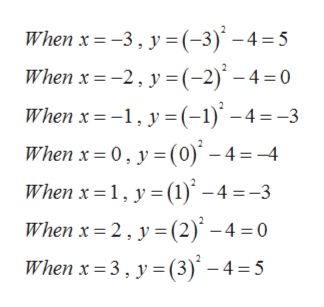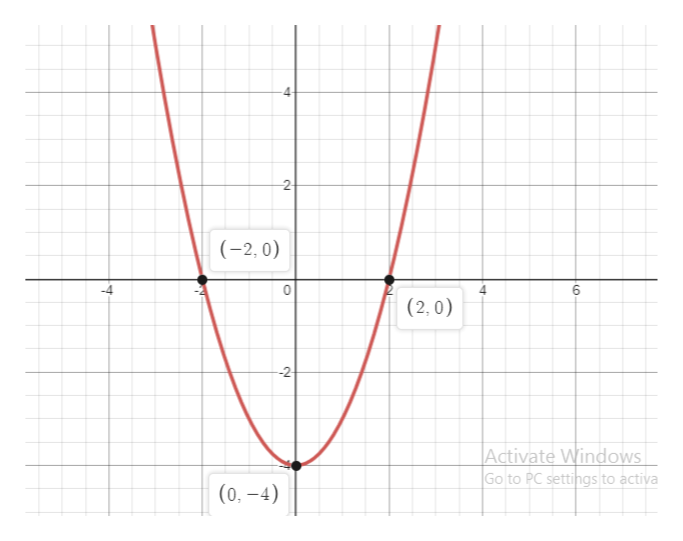Graph the given equation. First plot the solutions of the equation for the given values of x, and then connect the points with a smooth graph.y = x2 − 4x = −3, −2, −1, 0, 1, 2, 3 Graph the given equation. First plot the solutions of the equation for the given values of x, and then connect the points with a smooth graph.y = −x2 + 2x + 3x = −2, −1, 0, 1, 2, 3, 4

Question

Graph the given equation. First plot the solutions of the equation for the given values of x, and then connect the points with a smooth graph.

 y = x2 − 4 x = −3, −2, −1, 0, 1, 2, 3

Graph the given equation. First plot the solutions of the equation for the given values of x, and then connect the points with a smooth graph.

 y = −x2 + 2x + 3 x = −2, −1, 0, 1, 2, 3, 4

Step 1

Given,

Step 2

Calculating the values of y for the given values of x, we gethelp_outlineImage TranscriptioncloseWhen x -3, y -3)'-4 =5 When x -2,y(-2) -4 =0 When x 1,y(-1) -4=-3 When x 0, y(0) -4=4 When x 1 y() -4=-3 When x 2, y (2) -4 =0 When x 3. y (3)' -4=5 fullscreen
Step 3

The graph is,

&n...help_outlineImage Transcriptionclose4 2 (-2, 0) 6 4 (2,0) -2 Activate Windows Go to PC settings to activa (0,-4) fullscreen

Want to see the full answer?

See Solution

Want to see this answer and more?

Our solutions are written by experts, many with advanced degrees, and available 24/7

See Solution
Tagged in

Other